# (1 point) Consider a spring attached to a 1 kg mass, damping constant 6 = 9,...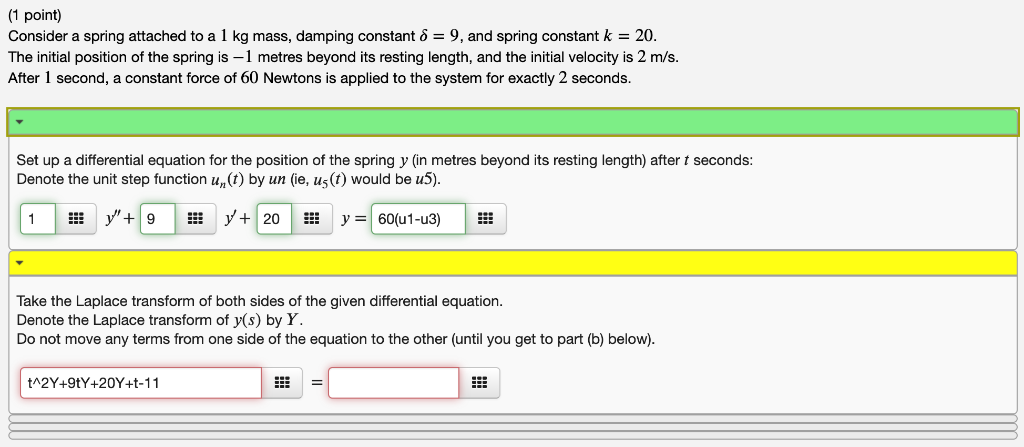(1 point) Consider a spring attached to a 1 kg mass, damping constant 6 = 9, and spring constant k = 20. The initial position of the spring is -1 metres beyond its resting length, and the initial velocity is 2 m/s. After 1 second, a constant force of 60 Newtons is applied to the system for exactly 2 seconds. Set up a differential equation for the position of the spring y (in metres beyond its resting length) after t seconds: Denote the unit step function un(t) by un (ie, us(t) would be u5). y"9 y20 y 60(u1-u3) 1 Take the Laplace transform of both sides of the given differential equation. Denote the Laplace transform of y(s) by Y. Do not move any terms from one side of the equation to the other (until you get to part (b) below). tA2Y+9tY+20Y+t-11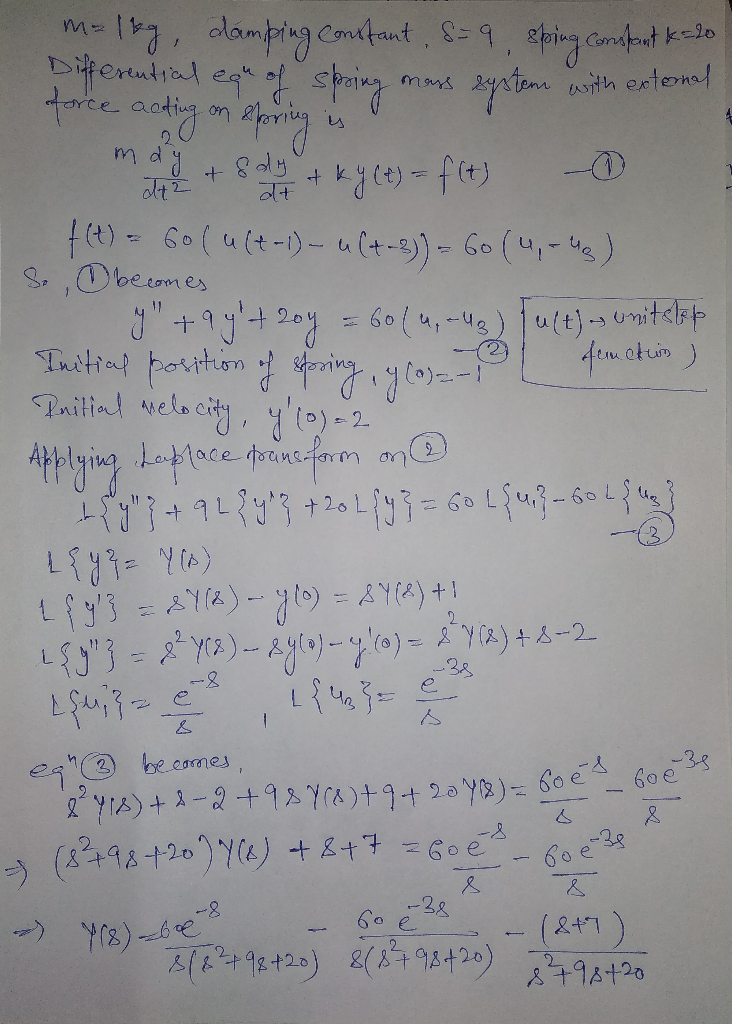#### Earn Coin

Coins can be redeemed for fabulous gifts.

Similar Homework Help Questions
• ### find the general solution (y) using laplace transform (1 point) Consider a spring attached to a...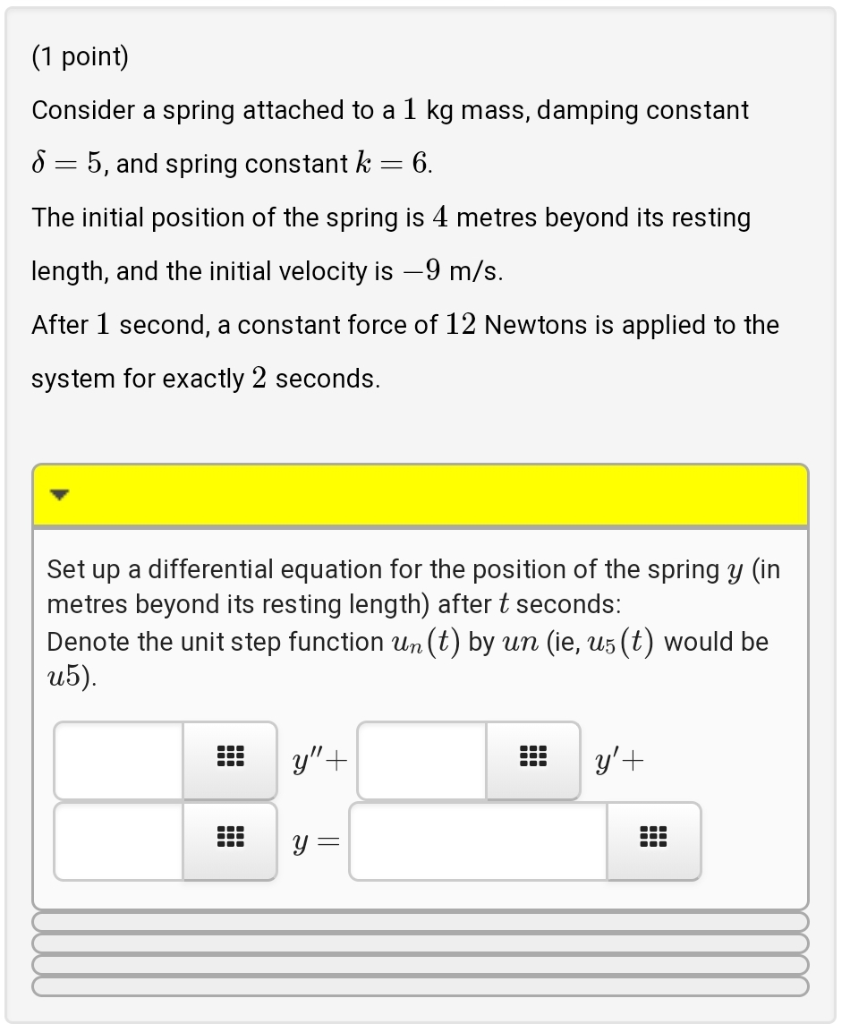find the general solution (y) using laplace transform (1 point) Consider a spring attached to a 1 kg mass, damping constant 8 = 5, and spring constant k = 6 The initial position of the spring is 4 metres beyond its resting length, and the initial velocity is -9 m/s. After 1 second, a constant force of 12 Newtons is applied to the system for exactly 2 seconds Set up a differential equation for the position of the spring y...

• ### (1 point) A spring-mass system with a 5-kg mass and a damping constant 8-N sec/m can...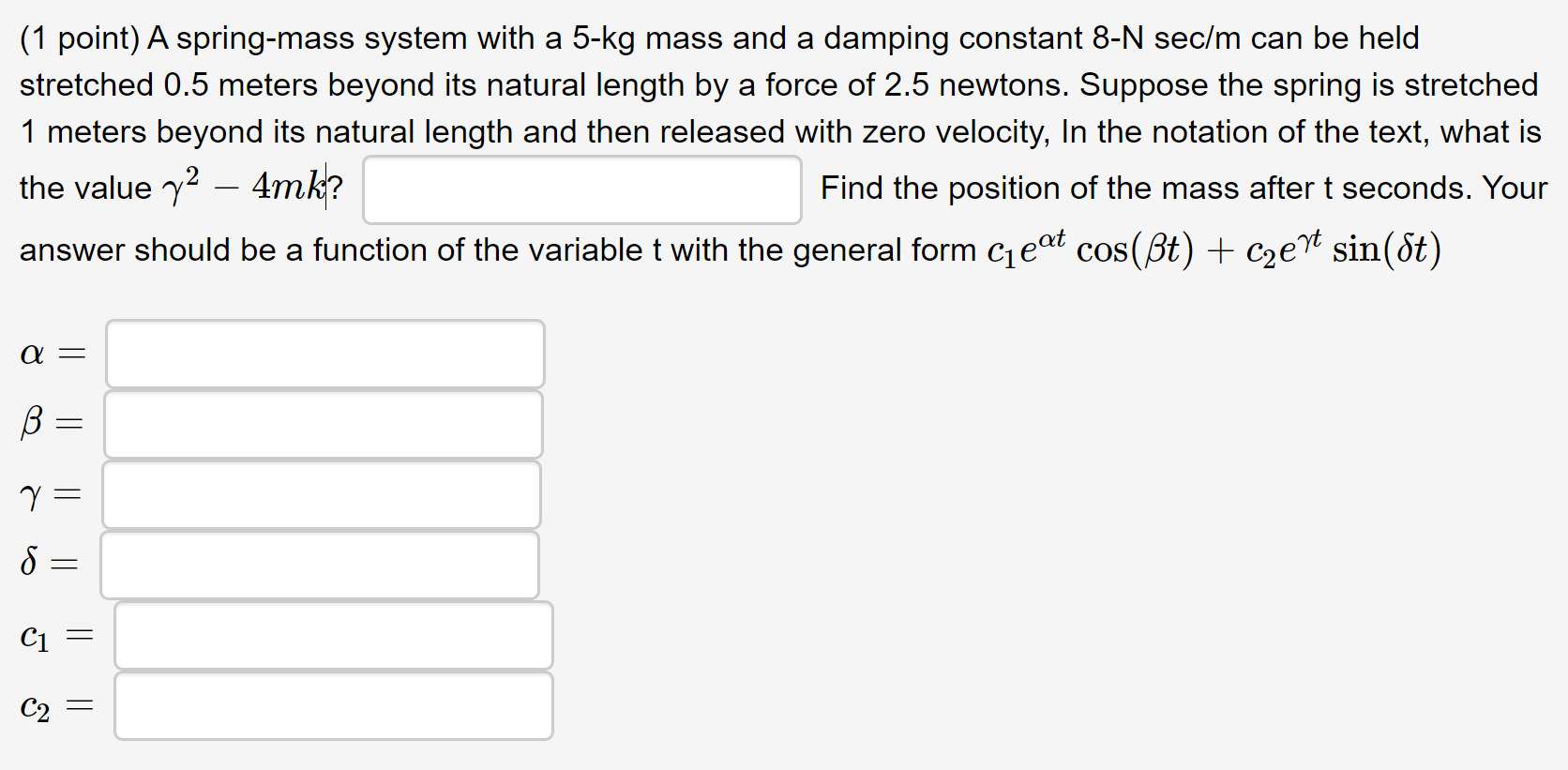(1 point) A spring-mass system with a 5-kg mass and a damping constant 8-N sec/m can be held stretched 0.5 meters beyond its natural length by a force of 2.5 newtons. Suppose the spring is stretched 1 meters beyond its natural length and then released with zero velocity, In the notation of the text, what is the value y2 – 4mk? Find the position of the mass after t seconds. Your answer should be a function of the variable t...

• ### Ignore damping forces. A mass of 4 kg is attached to a spring with constant k- 16 N/m, then the s...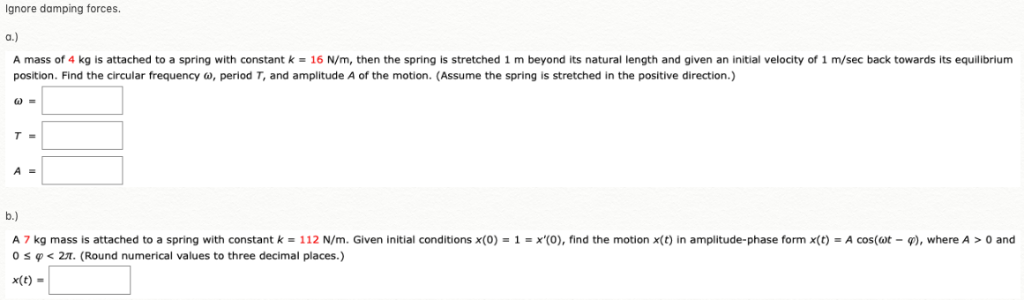Ignore damping forces. A mass of 4 kg is attached to a spring with constant k- 16 N/m, then the spring is stretched 1 m beyond its natural length and given an initial velocity of 1 m/sec back towards its equilibrium position. Find the circular frequency ω, period T, and amplitude A of the motion. (Assume the spring is stretched in the positive direction.) A 7 kg mass is attached to a spring with constant k 112 N m. Given...

• ### 2. A mass of m kilograms (kg) is mounted on top of a vertical spring. The spring is L metres long when disengaged and the end not attached to the mass is fixed to the ground. The mass moves verticall...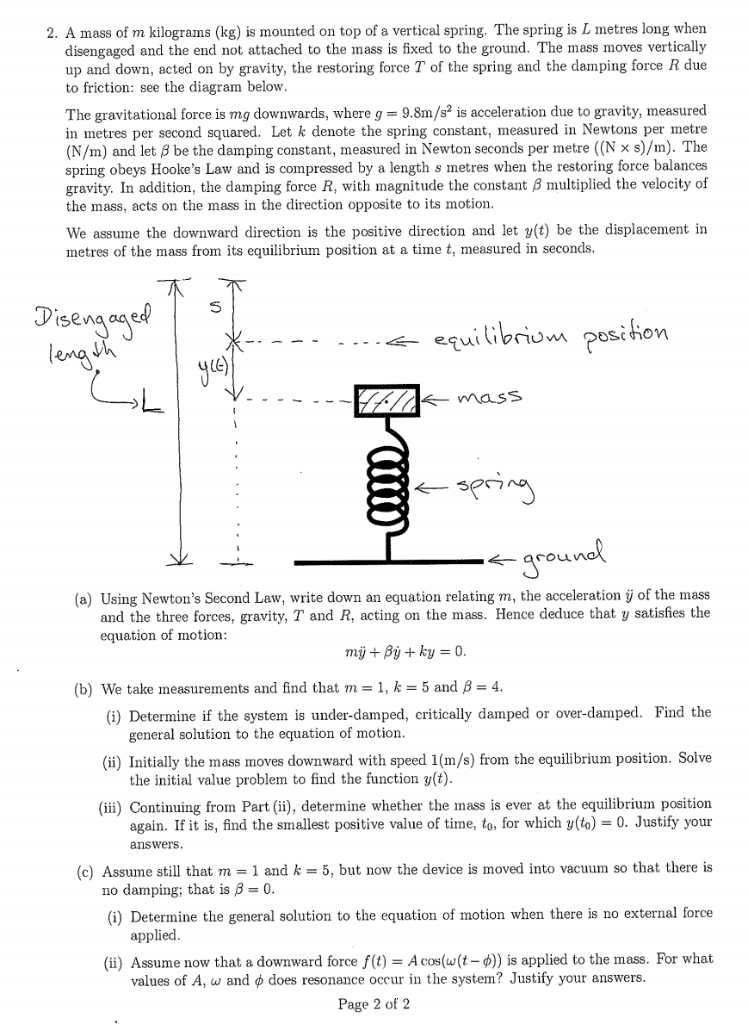2. A mass of m kilograms (kg) is mounted on top of a vertical spring. The spring is L metres long when disengaged and the end not attached to the mass is fixed to the ground. The mass moves vertically up and down, acted on by gravity, the restoring force T of the spring and the damping force R due to friction: see the diagram below The gravitational force is mg downwards, where g 9.8m/s is acceleration due to gravity,...

• ### 2. A mass of m kilograms (kg) is mounted on top of a vertical spring. The spring is L metres long when disengaged and the end not attached to the mass is fixed to the ground. The mass moves verticall...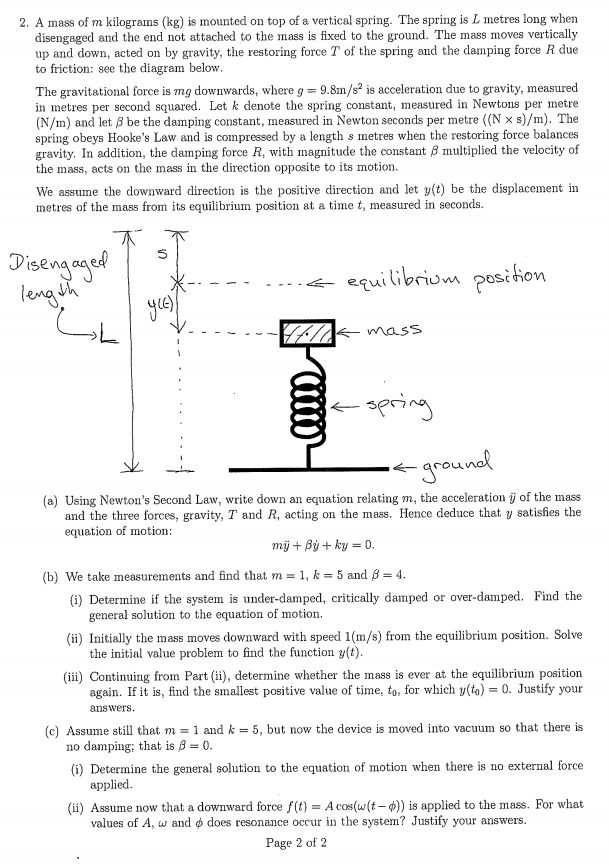2. A mass of m kilograms (kg) is mounted on top of a vertical spring. The spring is L metres long when disengaged and the end not attached to the mass is fixed to the ground. The mass moves vertically up and down, acted on by gravity, the restoring force T of the spring aud the damping force R due to friction: see the diagram below The gravitational force is mg downwards, where g 9.8m/s2 is acceleration due to gravity,...

• ### A mass of m kilograams (kg) is mounted on top of a vertical spring. The spring is L metres long when disengaged and the end not attached to the mass is fixced to the ground. The mass moves vertic...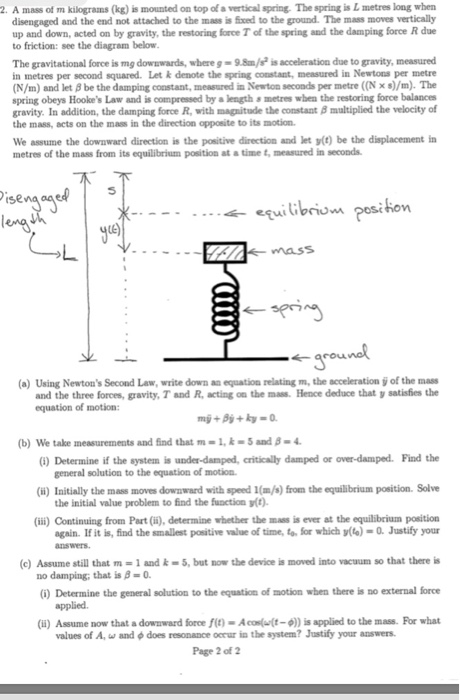A mass of m kilograams (kg) is mounted on top of a vertical spring. The spring is L metres long when disengaged and the end not attached to the mass is fixced to the ground. The mass moves vertically up and down, acted on by gravity, the restoring force T of the spring and the damping force R due to friction: see the diagram below The gravitational force is mg dowswards, where g- 9.8m is acceleration due to gravity, measured...

• ### An object of mass 4 grams hanging at the bottom of a spring with a spring...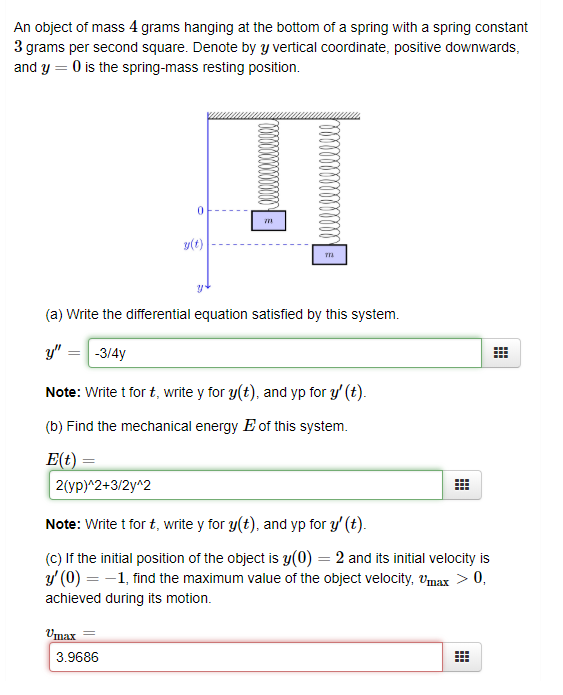An object of mass 4 grams hanging at the bottom of a spring with a spring constant 3 grams per second square. Denote by y vertical coordinate, positive downwards and y 0 is the spring-mass resting position. TTA (a) Write the differential equation satisfied by this system Note: Write t for t, write y for y(t), and yp for y' (t). (b) Find the mechanical energy E of this system. 2(yp)2+3/2y 2 Note: Write t for t, write y for...

• ### Ažkg mass is attached to a spring with stiffness 60 N/m. The damping constant for the...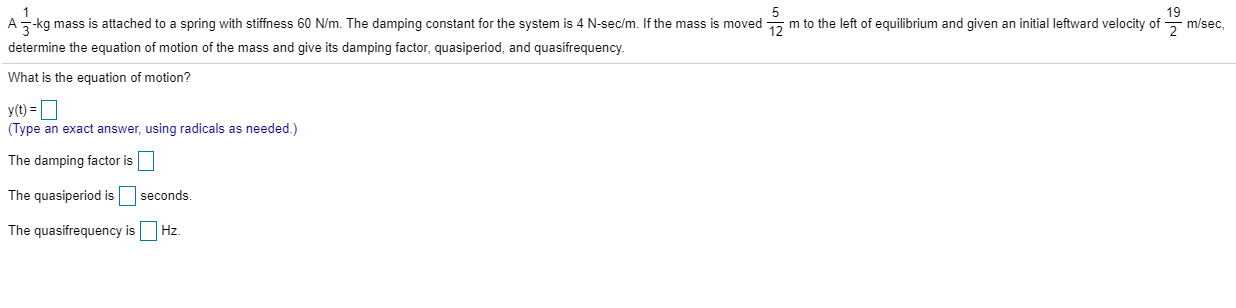Ažkg mass is attached to a spring with stiffness 60 N/m. The damping constant for the system is 4 N-sec'm. If the mass is moved a m to the left of equilibrium and given an initial leftward velocity of 19 2 m/sec determine the equation of motion of the mass and give its damping factor, quasiperiod, and quasifrequency What is the equation of motion? y(t) = 1 (Type an exact answer, using radicals as needed.) The damping factor is The...

• ### 8 1 A--kg mass is attached to a spring with stiffness 20 N/m. The damping constant...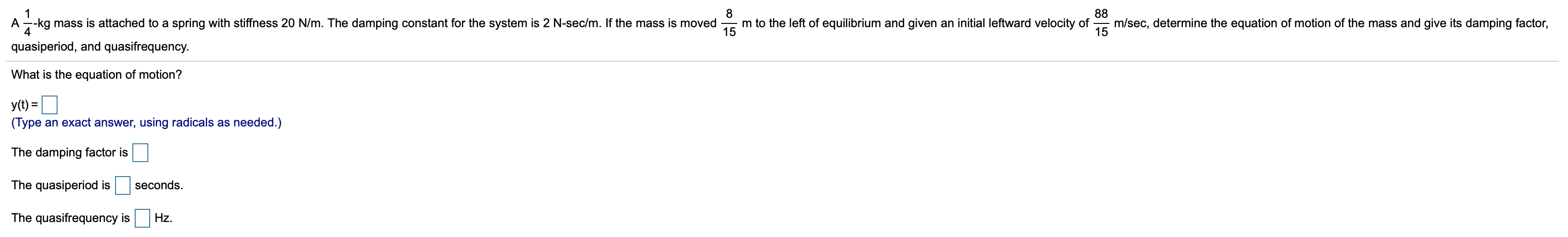8 1 A--kg mass is attached to a spring with stiffness 20 N/m. The damping constant for the system is 2 N-sec/m. If the mass is moved 4 quasiperiod, and quasifrequency. m/sec, determine the equation of motion of the mass and give its damping factor, 88 m to the left of equilibrium and given an initial leftward velocity of 15 15 What is the equation of motion? y(t) = (Type an exact answer, using radicals as needed.) The damping factor...

• ### A-kg mass is attached to a spring with stiffness 40 N/m. The damping constant for the...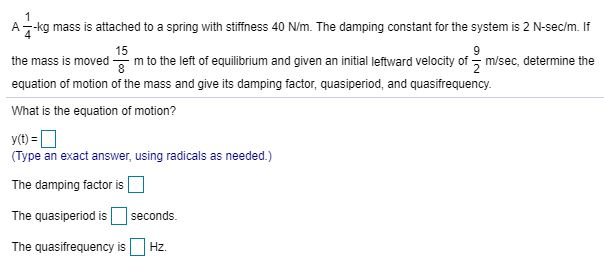A-kg mass is attached to a spring with stiffness 40 N/m. The damping constant for the system is 2 N-sec/m. If 15 the mass is moved 8 - m to the left of equilibrium and given an initial leftward velocity of į m/sec, determine the equation of motion of the mass and give its damping factor, quasiperiod, and quasifrequency. What is the equation of motion? y(t) = (Type an exact answer, using radicals as needed.) The damping factor is The...